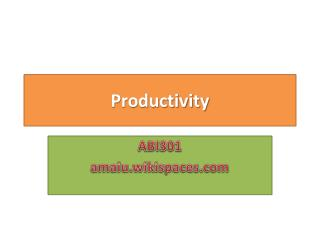# Productivity - PowerPoint PPT PresentationDownload PresentationProductivity

ProductivityDownload Presentation## Productivity

- - - - - - - - - - - - - - - - - - - - - - - - - - - E N D - - - - - - - - - - - - - - - - - - - - - - - - - - -
##### Presentation Transcript

1. Productivity ABI301 amaiu.wikispaces.com

2. Productivity Rules

3. Productivity Growth

4. Computing Productivity

5. Examples – Partial Productivity

6. Examples

7. Advantages for Productivity Measures • Productivity Measures are useful to the following: • To track performance over time • To determine what has changed and then devise means of improving productivity in the subsequent periods. • To judge the performance of an entire industry or the productivity of a country as a whole.

8. Factors that Affect Productivity: • Methods: by Standardizing the process and procedures where ever possible to reduce variability can have a significant benefit for both production and quality. • Capital: From manual packing to automated packing but requires capital investment for auto packing comparing to manual. • Quality: Example will it be 100% inspection which is time consuming or just random sampling? • Technology: the fact is that many productivity gains in the past have come from technological change such as automation, computers, calculators and so on…

9. Factors that Affect Productivity: • Management: A supportive management will boost morale of employees • Raw Materials: Will production be able to perform will if raw materials are substandard or don’t come on time? • Equipment: What will be the output if the machines are too old and experience downtime? • Working Condition/Environment: Will one produce more if the work area is too hot?

10. SAMPLE PROBLEMS FOR PRODUCTIVITY • Example # 1 • A company that processes fruits and vegetables is able to produce 400 cases of canned peaches in one half hour with four workers. What is the labor productivity? Productivity = output / labor hours worked = 400 / (4 x ½) = 400 / 2 = 200 Can per hour

11. SAMPLE PROBLEMS FOR PRODUCTIVITY • Example # 2 • A wrapping paper company produced 2,000 rolls of paper one day. Standard price is \$ 1/roll. Labor cost was \$ 160, material cost was \$ 50, and overhead was \$ 320. Determine the multifactor productivity. Productivity = output / (labor cost + material cost + overhead) = 2000 / (160+50+320) = 2000 / 530 = 3.77 rolls per \$

12. SAMPLE PROBLEMS FOR PRODUCTIVITY • Example # 3 • Find the productivity if four workers installed 720 square yards of carpeting in eight hours. Productivity = output / workers hours worked = 720 / (4 x 8) = 720 / 32 = 22.5 square yards per hour

13. SAMPLE PROBLEMS FOR PRODUCTIVITY • Example # 4 • Compute the productivity of a machine which produced 68 usable pieces in two hours. Productivity = output / machine hours produced = 68 / (1 x 2) = 68 / 2 = 34 usable pieces per hour

14. SAMPLE PROBLEMS FOR PRODUCTIVITY • Example # 5 • Determine the multifactor productivity for the combined input of the labor and the machine time using the following: • Input: • Labor: \$ 1,000; Materials: \$ 520; Overheads: \$ 2,000. Keep in mind the Production is 1760 unit Multifactor Productivity = output / (labor + materials + overheads) = 1760 / (1000 + 520 + 2000) = 1760 / 3520 = 0.5 unit per \$

15. SAMPLE PROBLEMS FOR PRODUCTIVITY Problem No#1 • Collins Little Company has a stuff of 4, each working 8 hours per day (for a payroll cost of \$ 640 / day) and overhead expenses of \$ 400 / day. Collins processes and closes on 8 titles each day. • The company recently purchased a computerized title search system that will allow the processing of 14 titles per day. Although the staff, their works hours, and pay will be same, the overheads expenses are now \$ 800 per day. Calculate: • Old labor productivity • New labor productivity • Old multifactor productivity • New multifactor productivity

16. Solution Part (a): Old labor productivity = output / input = 8 / (4 x 8) = 8 / 32 = 0.25 titles per labor hour Part (b): New labor productivity = output / input = 14 / (4 x 8) = 14 / 32 = 0.4375 titles per labor hour

17. Solution Part (c): Old multifactor productivity = output / (payroll + overhead) = 8 / (640 + 400) = 8 / 1040 = 0.0077 title/\$ Part (d): New multifactor productivity = output / (payroll + overhead) = 14 / (640 + 800) = 14 / 1440 = 0.0097 title/\$